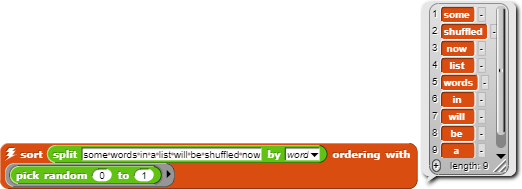# Reverse a list without the list OF

It turns out that SORT in the List Utilities library, when given a TRUE in the predicate slot, reverses the list it's given as an input:

Please tell me if there's a better category for this. (If there was a category for things users discover about Snap!, I would put this in it.)

Nice catch, but impractical, since sorting is slower than ordinary reversing.

I wonder if randomly choosing True or False would reliably shuffle the list.

It does!Yep. I tried it before I posted this topic, and I used exactly the same script as @helicoptur, but with my "moew esax asdf qwertyuiop" instead of his "some words in a list will be shuffled now".

The deeper question is whether all possible results come out with equal probability. (I don't know the answer, but I do know that some candidate shuffling algorithms are, e.g., weighted in favor of the first input item ending up near the front of the result.)

Hmm... The first item has a 50% chance of staying, no matter the length, so it probably isn't. I wonder if sort randomly -> reverse -> sort randomly works.

Better yet, have $$Probability\space of\space swap = {1\over len(list)}$$.

Well, for each item, it seems like it would be just as random as the "pick random" block is.

That would favor the extremes against the middle, no?

I think you're both making assumptions about the sort algorithm, namely that it's an insertion sort.

I think it would still favor extremes at the ends, though less so.

I think the sorting algorithm (that I don't know the name of) checks the predicate with each pair of items, and if it returns True, it swaps.

I may be totally wrong.

That's called "bubble sort," and is unlikely to be the algorithm used in the JS sort function (which is what we use), because bubble sort is $$O(n^2)$$ time. They probably use quicksort, which (ugh, hard to explain in one sentence) picks one random item and compares all the others against that one, dividing the list into the ones less than it and the ones more than it, then recursively sorts the two parts.

I think quicksort uses merge sort (what you explained) for lists of length > some value, and either insertion sort or selection sort for lists of length <= that value.

picks one random item

It might not be random, but I'm not sure.

On a StackOverflow article:

Numeric arrays ... are sorted using the C++ standard library function std::qsort which implements some variation of quicksort (usually introsort).

... Non-numeric arrays are stringified and sorted using mergesort ... or qsort if no merge sort is available.

For other types ... WebKit uses either selection sort ... or, in some cases, it sorts via an AVL tree.

Geez, pick a lane, JavaScript.

No, quicksort is entirely different from mergesort. It's a kind of partition sort with a bunch of little tweaks to improve efficiency. Check out https://snap.berkeley.edu/snap/snap.html#present:Username=bh&ProjectName=sorting to see four different sort algorithms including partition sort.

@helicoptur I wonder why they use quicksort for numbers but mergesort for strings. Must be something about the variable-width items. And I'm really curious as to why they'd ever use selection sort, unless the number of items is small.